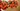# 理解 JavaScript 的柯里化

• 纯函数
• 柯里化
• 高阶函数

## 什么是柯里化？

``````function fn(a, b) {
//...
}

function _fn(a, b, c) {
//...
}``````

``````function multiply(a, b, c) {
return a * b * c;
}``````

``multiply(1, 2, 3); // 6``

``````function multiply(a) {
return (b) => {
return (c) => {
return a * b * c;
};
};
}
log(multiply(1)(2)(3)); // 6``````

``````const mul1 = multiply(1);
const mul2 = mul1(2);
const result = mul2(3);
log(result); // 6``````

``let mul1 = multiply(1);``

``````return (b) => {
return (c) => {
return a * b * c;
};
};``````

``let mul2 = mul1(2);``

num1 会返回第三个参数：

``````return (c) => {
return a * b * c;
};``````

`mul2` 会变成：

``````mul2 = (c) => {
return a * b * c;
};``````

``const result = mul2(3);``

``log(result); // 6``

``````function volume(l, w, h) {
return l * w * h;
}
const aCylinder = volume(100, 20, 90); // 180000``````

``````function volume(l) {
return (w) => {
return (h) => {
return l * w * h;
};
};
}
const aCylinder = volume(100)(20)(90); // 180000``````

## 数学中的柯里化

``f(x, y) = x^2 + y = z``

``f(x,y) = f(3, 4) = x^2 + y = 3^2 + 4 = 13 = z``

``````h = x^2 + y = f(x, y)
hy(x) = x^2 + y = hx(y) = x^2 + y

[hx => w.r.t x] and [hy => w.r.t y]``````

``````h3(y) = 3^2 + y = 9 + y

// Note: h3 is h subscript 3``````

``h3(y) = h(3)(y) = f(3, y) = 3^2 + y = 9 + y``

``h3(4) = h(3)(4) = f(3,4) = 9 + 4 = 13``

`y` 是这条链中的最后一个变量，加法操作会对它和依然存在的之前的变量 `x = 3` 做运算并得出结果，`13`

``````3^2 + y -> 9 + y

f(3, y) = h3(y) = 3^2 + y = 9 + y
f(3, y) = 9 + y
f(3, 4) = h3(4) = 9 + 4 = 13``````

Wow！！这是一些数学问题，如果你觉得不够清晰 😕。可以在 Wikipedia 查看 📖 完整的细节。

## 柯里化和部分函数应用

``````function volume(l) {
return (w, h) => {
return l * w * h;
};
}``````

``````const hCy = volume(70);
hCy(203, 142);
hCy(220, 122);
hCy(120, 123);``````

``````volume(70)(90, 30);
volume(70)(390, 320);
volume(70)(940, 340);``````

``````function acidityRatio(x, y, z) {
return performOp(x, y, z);
}
// |
// V
function acidityRatio(x) {
return (y, z) => {
return performOp(x, y, z);
};
}``````

``````function acidityRatio(x) {
return (y) => {
return (z) => {
return performOp(x, y, z);
};
};
}``````

``````function div(x, y) {
return x / y;
}``````

``````function div(x) {
return (y) => {
return x / y;
};
}``````

``````function div(x) {
return (y) => {
return x / y;
};
}``````

## 柯里化有用吗？

1. 编写小模块的代码，可以更轻松的重用和配置，就像 npm 做的那样：

``````function discount(price, discount) {
return price * discount;
}``````

``````const price = discount(500, 0.1); // \$50
// \$500  - \$50 = \$450``````

``````const price = discount(1500, 0.1); // \$150
// \$1,500 - \$150 = \$1,350
const price = discount(2000, 0.1); // \$200
// \$2,000 - \$200 = \$1,800
const price = discount(50, 0.1); // \$5
// \$50 - \$5 = \$45
const price = discount(5000, 0.1); // \$500
// \$5,000 - \$500 = \$4,500
const price = discount(300, 0.1); // \$30
// \$300 - \$30 = \$270``````

``````function discount(discount) {
return (price) => {
return price * discount;
};
}

const tenPercentDiscount = discount(0.1);``````

``````tenPercentDiscount(500); // \$50
// \$500 - \$50 = \$450``````

``const twentyPercentDiscount = discount(0.2);``

``````twentyPercentDiscount(500); // 100
// \$500 - \$100 = \$400
twentyPercentDiscount(5000); // 1000
// \$5,000 - \$1,000 = \$4,000
twentyPercentDiscount(1000000); // 200000
// \$1,000,000 - \$200,000 = \$600,000``````

2. 避免频繁调用具有相同参数的函数：

``````function volume(l, w, h) {
return l * w * h;
}``````

``````volume(200, 30, 100); // 2003000l
volume(32, 45, 100); // 144000l
volume(2322, 232, 100); // 53870400l``````

``````function volume(h) {
return (w) => {
return (l) => {
return l * w * h;
};
};
}``````

``````const hCylinderHeight = volume(100);
hCylinderHeight(200)(30); // 600,000l
hCylinderHeight(2322)(232); // 53,870,400l``````

## 通用的柯里化函数

``````function curry(fn, ...args) {
return (..._arg) => {
return fn(...args, ..._arg);
};
}``````

``````function volume(l, h, w) {
return l * h * w;
}
const hCy = curry(volume, 100);
hCy(200, 900); // 18000000l
hCy(70, 60); // 420000l``````